ML之4PolyR：利用四次多项式回归4PolyR模型+两种正则化(Lasso/Ridge)在披萨数据集上拟合(train)、价格回归预测(test)

+关注继续查看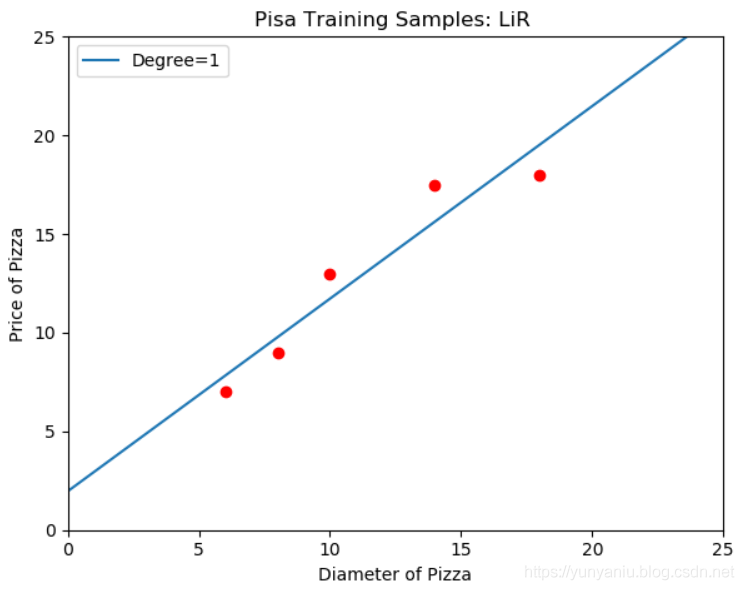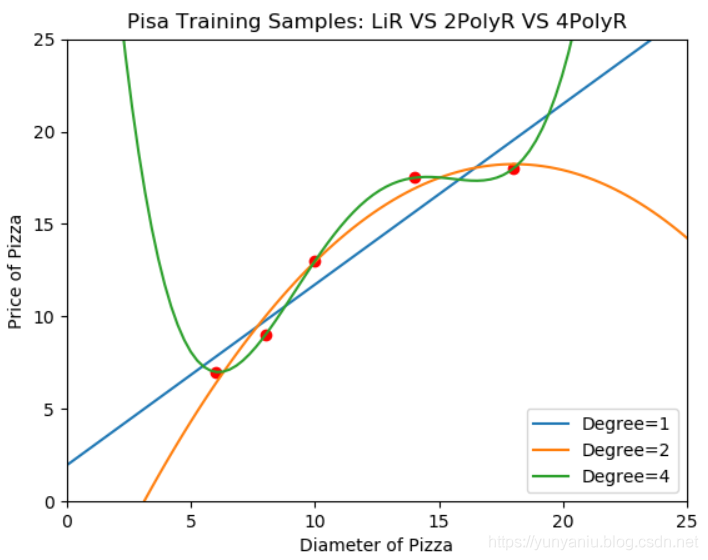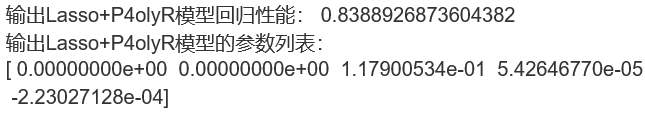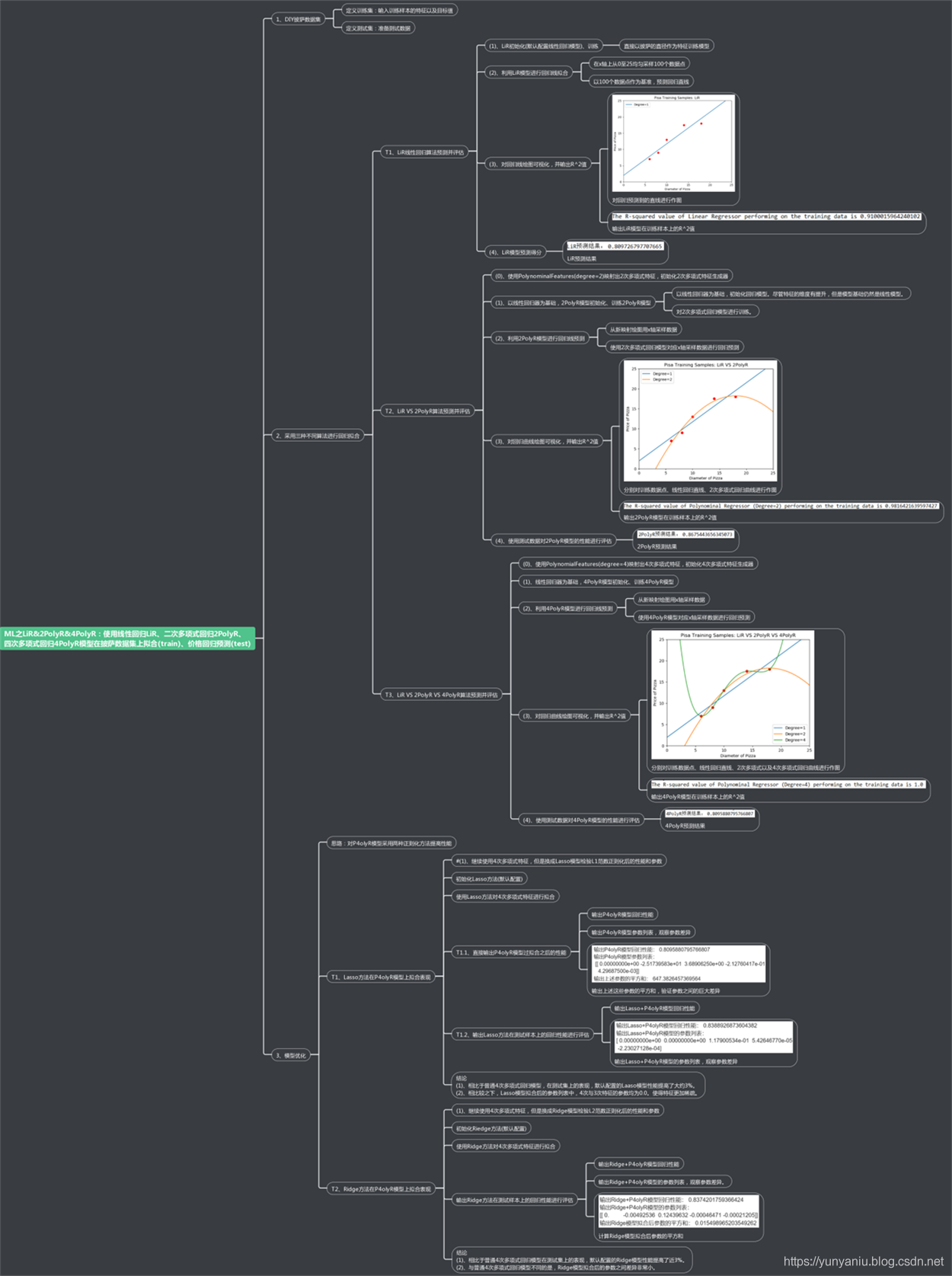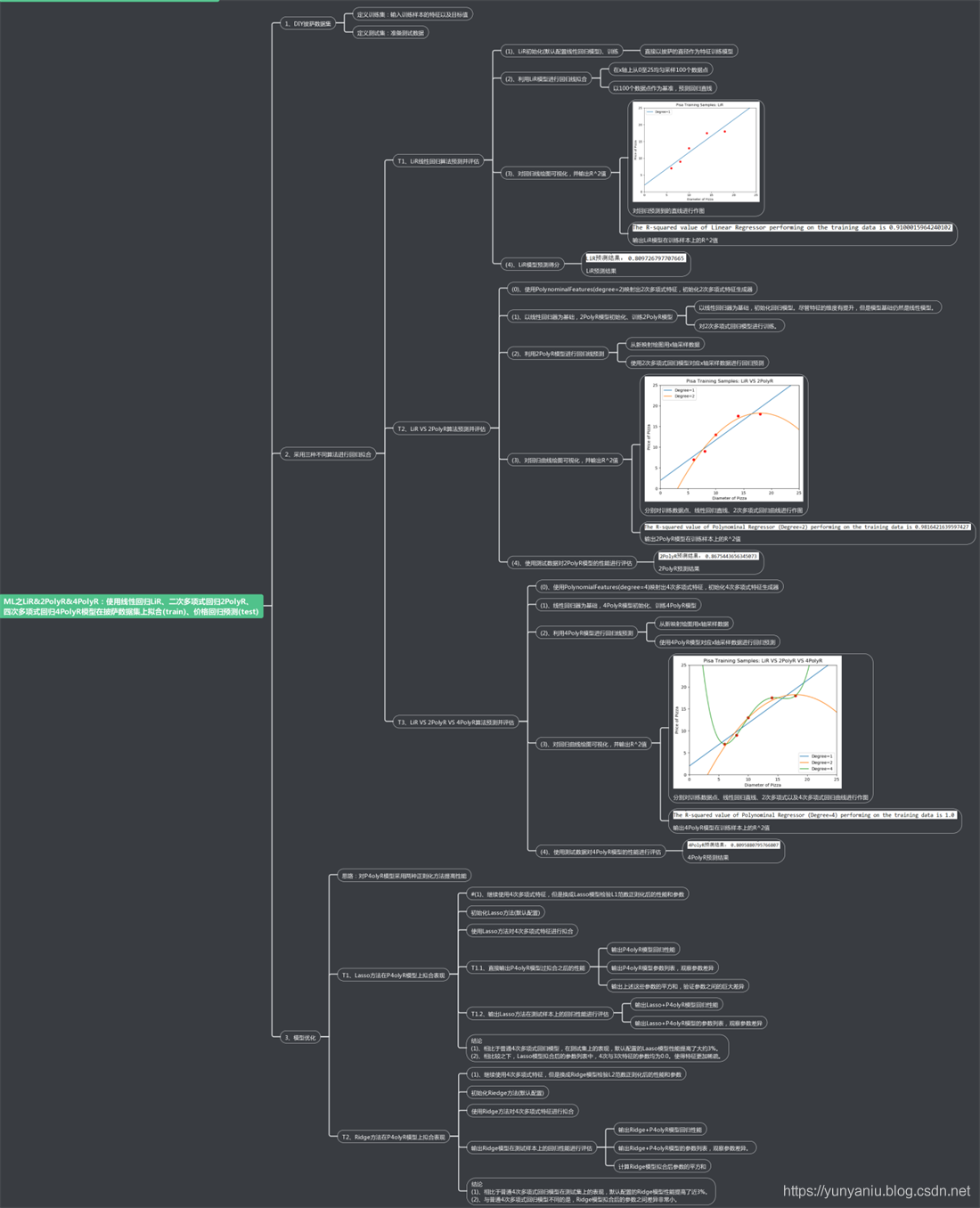lasso_poly4 = Lasso()

lasso_poly4.fit(X_train_poly4, y_train)

ridge_poly4 = Ridge()

ridge_poly4.fit(X_train_poly4, y_train)

class Lasso(ElasticNet):

"""Linear Model trained with L1 prior as regularizer (aka the Lasso)

The optimization objective for Lasso is::

(1 / (2 * n_samples)) * ||y - Xw||^2_2 + alpha * ||w||_1

Technically the Lasso model is optimizing the same objective function as

the Elastic Net with l1_ratio=1.0 (no L2 penalty).

Read more in the :ref:User Guide <lasso>.

Parameters

----------

alpha : float, optional

Constant that multiplies the L1 term. Defaults to 1.0.

alpha = 0 is equivalent to an ordinary least square, solved

by the :class:LinearRegression object. For numerical

reasons, using alpha = 0 with the Lasso object is not advised.

Given this, you should use the :class:LinearRegression object.

fit_intercept : boolean

whether to calculate the intercept for this model. If set

to false, no intercept will be used in calculations

(e.g. data is expected to be already centered).

normalize : boolean, optional, default False

This parameter is ignored when fit_intercept is set to False.

If True, the regressors X will be normalized before regression by

subtracting the mean and dividing by the l2-norm.

If you wish to standardize, please use

:class:sklearn.preprocessing.StandardScaler before calling fit

on an estimator with normalize=False.

precompute : True | False | array-like, default=False

Whether to use a precomputed Gram matrix to speed up

calculations. If set to 'auto' let us decide. The Gram

matrix can also be passed as argument. For sparse input

this option is always True to preserve sparsity.

copy_X : boolean, optional, default True

If True, X will be copied; else, it may be overwritten.

max_iter : int, optional

The maximum number of iterations

tol : float, optional

The tolerance for the optimization: if the updates are

smaller than tol, the optimization code checks the

dual gap for optimality and continues until it is smaller

than tol.

warm_start : bool, optional

When set to True, reuse the solution of the previous call to fit as

initialization, otherwise, just erase the previous solution.

positive : bool, optional

When set to True, forces the coefficients to be positive.

random_state : int, RandomState instance or None, optional, default

None

The seed of the pseudo random number generator that selects a

random

feature to update.  If int, random_state is the seed used by the random

number generator; If RandomState instance, random_state is the

random

number generator; If None, the random number generator is the

RandomState instance used by np.random. Used when selection ==

'random'.

selection : str, default 'cyclic'

If set to 'random', a random coefficient is updated every iteration

rather than looping over features sequentially by default. This

(setting to 'random') often leads to significantly faster convergence

especially when tol is higher than 1e-4.

Attributes

----------

coef_ : array, shape (n_features,) | (n_targets, n_features)

parameter vector (w in the cost function formula)

sparse_coef_ : scipy.sparse matrix, shape (n_features, 1) | \

(n_targets, n_features)

sparse_coef_ is a readonly property derived from coef_

intercept_ : float | array, shape (n_targets,)

independent term in decision function.

n_iter_ : int | array-like, shape (n_targets,)

number of iterations run by the coordinate descent solver to reach

the specified tolerance.

Examples

--------

>>> from sklearn import linear_model

>>> clf = linear_model.Lasso(alpha=0.1)

>>> clf.fit([[0,0], [1, 1], [2, 2]], [0, 1, 2])

Lasso(alpha=0.1, copy_X=True, fit_intercept=True, max_iter=1000,

normalize=False, positive=False, precompute=False,

random_state=None,

selection='cyclic', tol=0.0001, warm_start=False)

>>> print(clf.coef_)

[ 0.85  0.  ]

>>> print(clf.intercept_)

0.15

--------

lars_path

lasso_path

LassoLars

LassoCV

LassoLarsCV

sklearn.decomposition.sparse_encode

Notes

-----

The algorithm used to fit the model is coordinate descent.

To avoid unnecessary memory duplication the X argument of the fit

method

should be directly passed as a Fortran-contiguous numpy array.

"""

path = staticmethod(enet_path)

def __init__(self, alpha=1.0, fit_intercept=True, normalize=False,

precompute=False, copy_X=True, max_iter=1000,

tol=1e-4, warm_start=False, positive=False,

random_state=None, selection='cyclic'):

super(Lasso, self).__init__(alpha=alpha, l1_ratio=1.0,

fit_intercept=fit_intercept, normalize=normalize,

precompute=precompute, copy_X=copy_X, max_iter=max_iter, tol=tol,

warm_start=warm_start, positive=positive, random_state=random_state,

selection=selection)

######################################################

#########################

# Functions for CV with paths functions【技术干货】想要高效采集数据到阿里云Elasticsearch，这些方法你知道吗？

3247 01029 0PowerDesigner PDM表数据信息用表格展现

643 010886 0SharePoint 2013 Designer系列之数据视图筛选
在SharePoint中，我们经常需要对列表进行简单的筛选，这时，数据视图就有作用了，我们可以定制对于字段的筛选，来进行展示；特别的，筛选不同于搜索，并没有对于附件或者文档的全文检索，如果需要全文检索，可以使用列表的垂直搜索功能。
731 0PowerDesigner教程系列（六）概念数据模型

700 0SharePoint 2013 Designer系列之数据视图
在SharePoint使用中，数据展示是一块很重要的部分，很多时候我们会采用webpart的形式，但是有一些情况，我们不必使用开发，仅需使用Designer即可，下面让我简单介绍下数据视图的使用。 　　1、创建一个测试列表，以下为测试列表的字段，如下图： 　　2、插入一些测试数据（纯属捏...
668 0C语言数据结构-稀疏多项式运算

4 03551 0

1701

0

《2021云上架构与运维峰会演讲合集》

《零基础CSS入门教程》

《零基础HTML入门教程》